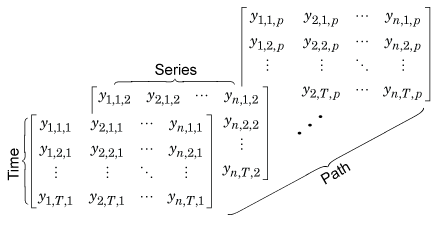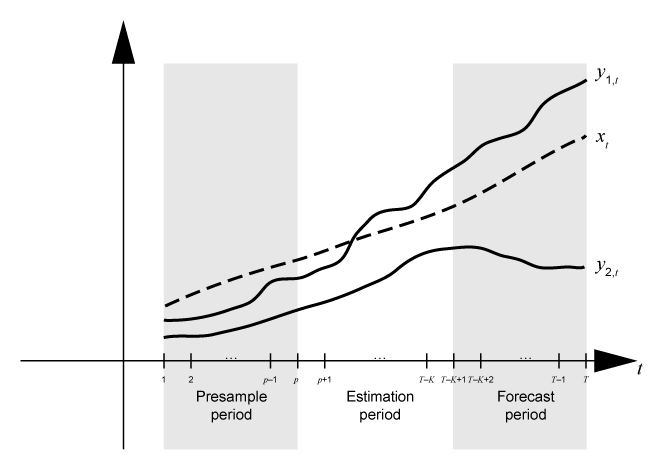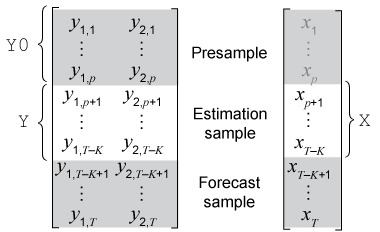Main Content

## Multivariate Time Series Data Formats

The first step in multivariate time series analysis is to obtain, inspect, and preprocess data. This topic describes the following:

• How to load economic data into MATLAB®

• Appropriate data types and structures for multivariate time series analysis functions

• Common characteristics of time series data that can warrant transforming the set before proceeding with an analysis

• How to partition your data into presample, estimation, and forecast samples.

### Multivariate Time Series Data

Two main types of multivariate time series data are:

• Response data – Observations from the n-D multivariate time series of responses yt (see Types of Stationary Multivariate Time Series Models).

• Exogenous data – Observations from the m-D multivariate time series of predictors xt. Each variable in the exogenous data appears in all response equations by default.

Before specifying any data set as an input to Econometrics Toolbox™ functions, format the data appropriately. Use standard MATLAB commands, or preprocess the data with a spreadsheet program, database program, PERL, or other tool.

You can obtain historical time series data from several freely available sources, such as the St. Louis Federal Reserve Economics Database (known as FRED®): `https://research.stlouisfed.org/fred2/`. If you have a Datafeed Toolbox™ license, you can use the toolbox functions to access data from various sources.

### Load Multivariate Economic Data

The file `Data_USEconModel` ships with Econometrics Toolbox. It contains time series from FRED.

Load the data into the MATLAB Workspace.

`load Data_USEconModel`

Variables in the workspace include:

• `Data`, a 249-by-14 matrix containing 14 macroeconomic time series.

• `DataTable`, a 249-by-14 MATLAB timetable array containing timestamped data.

• `dates`, a 249-element vector containing MATLAB serial date numbers representing sampling dates. A serial date number is the number of days since January 1, 0000. (This "date" is not a real date, but is convenient for making date calculations. For more details, see Date Formats in the Financial Toolbox™ User's Guide.)

• `Description`, a character array containing a description of the data series and the key to the labels for each series.

• `series`, a 1-by-14 cell array of labels for the time series.

`DataTable` contains the same data as `Data`. However, like a table, a timetable enables you to use dot notation to access a variable. For example, `DataTable.UNRATE` specifies the unemployment rate time series. All timetables contain the variable `Time`, which is a `datetime` vector of observation timestamps. For more details, see Create Timetables and Represent Dates and Times in MATLAB. You can also work with the MATLAB serial date numbers stored in `dates`.

Display the first and last sampling times and the names of the variables by using `DataTable`.

`firstperiod = DataTable.Time(1)`
```firstperiod = datetime Q1-47 ```
`lastperiod = DataTable.Time(end)`
```lastperiod = datetime Q1-09 ```
`seriesnames = DataTable.Properties.VariableNames`
```seriesnames = 1x14 cell Columns 1 through 6 {'COE'} {'CPIAUCSL'} {'FEDFUNDS'} {'GCE'} {'GDP'} {'GDPDEF'} Columns 7 through 12 {'GPDI'} {'GS10'} {'HOANBS'} {'M1SL'} {'M2SL'} {'PCEC'} Columns 13 through 14 {'TB3MS'} {'UNRATE'} ```

This table describes the variables in `DataTable`.

FRED VariableDescription
`COE`Paid compensation of employees in \$ billions
`CPIAUCSL` Consumer price index (CPI)
`FEDFUNDS`Effective federal funds rate
`GCE`Government consumption expenditures and investment in \$ billions
`GDP`Gross domestic product (GDP)
`GDPDEF`Gross domestic product in \$ billions
`GPDI`Gross private domestic investment in \$ billions
`GS10`Ten-year treasury bond yield
`HOANBS`Nonfarm business sector index of hours worked
`M1SL `M1 money supply (narrow money)
`M2SL`M2 money supply (broad money)
`PCEC`Personal consumption expenditures in \$ billions
`TB3MS`Three-month treasury bill yield
`UNRATE`Unemployment rate

Consider studying the dynamics of the GDP, CPI, and unemployment rate, and suppose government consumption expenditures is an exogenous variable. Create arrays for the response and predictor data. Display the latest observation in each array.

```Y = DataTable{:,["CPIAUCSL" "UNRATE" "GDP"]}; x = DataTable.GCE; lastobsresponse = Y(end,:)```
```lastobsresponse = 1×3 104 × 0.0213 0.0008 1.4090 ```
`lastobspredictor = x(end)`
```lastobspredictor = 2.8833e+03 ```

`Y` and `x` represent one path of observations, and are appropriately formatted for passing to multivariate model object functions. The timestamp information does not apply to the arrays because analyses assume sampling times are evenly spaced.

### Multivariate Data Format

Usually, you load response and predictor data sets into the MATLAB Workspace as numeric arrays, MATLAB tables, or MATLAB timetables. However, multivariate time series object functions accept 2-D or 3-D numeric arrays only, and you must specify the response and predictor data as separate inputs.

The type of variable and problem context determine the format of the data that you supply. For any array containing multivariate time series data:

• Row t of the array contains the observations of all variables at time t.

• Column j of the array contains all observations of variable j. MATLAB treats each variable in an array as distinct.

A matrix of data indicates one sample path. To create a variable representing one path of length T of response data, put the data into a T-by-n matrix `Y`:

`$\left[\begin{array}{cccc}{y}_{1,1}& {y}_{2,1}& \cdots & {y}_{n,1}\\ {y}_{1,2}& {y}_{2,2}& \cdots & {y}_{n,2}\\ ⋮& ⋮& \ddots & ⋮\\ {y}_{1,T}& {y}_{2,T}& \cdots & {y}_{n,T}\end{array}\right].$`

`Y(t,j)` = yj,t, which is observation t of response variable j. A single path of data created from predictor variables, or other variables, has a similar form.

You can specify one path of observations as an input to all multivariate model object functions that accept data. Examples of situations in which you supply one path include:

A 3-D numeric array indicates multiple independent sample paths of data. You can create T-by-n-by-p array `Y`, representing p sample paths of response data, by stacking single paths of responses (matrices) along the third dimension.

``

`Y(t,j,k)` = yj,t,k, which is observation t of response variable j from path k, k = 1,…,p. All paths must have the same sample times, and variables among paths must correspond. For more details, see Multidimensional Arrays.

You can specify an array of multiple paths of responses or innovations as an input to several multivariate model object functions that accept data. Examples of situations in which you supply multiple paths include:

• Initialize a VEC model with multiple paths of presample data for forecasting or simulating multiple paths. Each specified path can represent different initial conditions, from which the functions generate forecasts or simulations.

• Obtain multiple response paths from filtering multiple paths of innovations through a VAR model. This process is an alternative way to simulate multiple response paths.

• Generate multiple conditional forecast paths from a VAR model given multiple paths of future response data.

`estimate` does not support the specification of multiple paths of response data.

#### Exogenous Data Format

All multivariate model object functions that take exogenous data as an input accept a matrix X representing one path of observations. MATLAB includes all exogenous variables in the regression component of each response equation. For a VAR(p) model, the response equations are:

`$\left[\begin{array}{c}{y}_{1,t}\\ {y}_{2,t}\\ ⋮\\ {y}_{n,t}\end{array}\right]=c+\delta t+\left[\begin{array}{c}{x}_{1,t}\beta \left(1,1\right)+\cdots +{x}_{m,t}\beta \left(1,m\right)\\ {x}_{1,t}\beta \left(2,1\right)+\cdots +{x}_{m,t}\beta \left(2,m\right)\\ ⋮\\ {x}_{1,t}\beta \left(n,1\right)+\cdots +{x}_{m,t}\beta \left(n,m\right)\end{array}\right]+\sum _{j=1}^{p}{\Phi }_{j}{y}_{t-j}+{\epsilon }_{t}.$`

To configure the regression components of the response equations, work with the regression coefficient matrix (stored in the `Beta` property of the model object) rather than the data. For more details, see Create VAR Model and Select Exogenous Variables for Response Equations.

Multivariate model object functions do not support multiple paths of predictor data. However, if you specify a path of predictor data and multiple paths of response or innovations data, the function associates the same predictor data to all paths. For example, if you simulate paths of responses from a VARX model and specify multiple paths of presample values, `simulate` applies the same exogenous data to each generated response path.

### Preprocess Data

Your data might have characteristics that violate model assumptions. For example, you can have data with exponential growth, or data from multiple sources at different periodicities. In such cases, preprocess or transform the data to an acceptable form for analysis.

• Inspect the data for missing values, which are indicated by `NaN`s. By default, object functions use list-wise deletion to remove observations containing at least one missing value. If at least one response or predictor variable has a missing value for a time point (row), MATLAB removes all observations for that time (the entire row of the response and predictor data matrices). Such deletion can have implications on the time base and the effective sample size. Therefore, you should investigate and address any missing values before starting an analysis.

• For data from multiple sources, you must decide how to synchronize the data. Data synchronization can include data aggregation or disaggregation, and the latter can create patterns of missing values. You can address these types of induced missing values by imputing previous values (that is, a missing value is unchanged from its previous value), or by interpolating them from neighboring values.

If the time series are variables in a timetable, then you can synchronize your data by using `synchronize`.

• For time series exhibiting exponential growth, you can preprocess the data by taking the logarithm of the growing series. In some cases, you must apply the first difference of the result (see `price2ret`). For more details on stabilizing time series, see Unit Root Nonstationarity. For an example, see VAR Model Case Study.

Note

If you apply the first difference of a series, the resulting series is one observation shorter than the original series. If you apply the first difference of only some time series in a data set, truncate the other series so that all have the same length, or pad the differenced series with initial values.

### Time Base Partitions for Estimation

When you fit a time series model to data, lagged terms in the model require initialization, usually with observations at the beginning of the sample. Also, to measure the quality of forecasts from the model, you must hold out data at the end of your sample from estimation. Therefore, before analyzing the data, partition the time base into three consecutive, disjoint intervals:

Three time base partitions for multivariate vector autoregression (VAR) and vector error-correction (VEC) models are the presample, estimation, and forecast periods.

• Presample period – Contains data used to initialize lagged values in the model. Both VAR(p) and VEC(p–1) models require a presample period containing at least p multivariate observations. For example, if you plan to fit a VAR(4) model, the conditional expected value of yt, given its history, contains yt – 1, yt – 2, yt – 3, and yt – 4. The conditional expected value of y5 is a function of y1, y2, y3, and y4. Therefore, the likelihood contribution of y5 requires y1y4, which implies that data does not exist for the likelihood contributions of y1y4. In this case, model estimation requires a presample period of at least four time points.

• Estimation period — Contains the observations to which the model is explicitly fit. The number of observations in the estimation sample is the effective sample size. For parameter identifiability, the effective sample size should be at least the number of parameters being estimated.

• Forecast period — Optional period during which forecasts are generated, known as the forecast horizon. This partition contains holdout data for model predictability validation.

Suppose yt is a 2-D response series and xt is a 1-D exogenous series. Consider fitting a VARX(p) model of yt to the response data in the T-by-2 matrix `Y` and the exogenous data in the T-by-1 vector `x`. Also, you want the forecast horizon to have length K (that is, you want to hold out K observations at the end of the sample to compare to the forecasts from the fitted model). This figure shows the time base partitions for model estimation.This figure shows portions of the arrays that correspond to input arguments of the `estimate` function.• `Y` is the required input for specifying the response data to which the model is fit.

• `Y0` is an optional name-value pair argument for specifying the presample response data. `Y0` must have at least p rows. To initialize the model, `estimate` uses only the latest p observations `Y0((end – p + 1):end,:)`.

• `X` is an optional name-value pair argument for specifying exogenous data for the linear regression component. By default, `estimate` excludes a regression component from the model, regardless of the value of the regression coefficient `Beta` of the `arima` model template for estimation.

If you do not specify `'Y0'`, `estimate` removes observations 1 through p from `Y` to initialize the model, and then fits the model to the rest of the data `Y((p + 1):end,:)`. That is, `estimate` infers the presample and estimation periods from `Y`. Although `estimate` extracts the presample from `Y` by default, you can extract the presample from the data and specify it using the `Y0` name-value pair argument, which ensures that `estimate` initializes and fits the model to your specifications.

If you specify `'X'`:

• `estimate` synchronizes `X` and `Y` with respect to the last observation in the arrays (TK in the previous figure), and applies only the required number of observations to the regression component. This action implies that `X` can have more rows that `Y`.

• If you also specify `'Y0'`, `estimate` uses only the latest exogenous observations required to fit the model (observations J + 1 through TK in the previous figure). `estimate` ignores presample exogenous data.

If you plan to validate the predictive power of the fitted model, you must extract the forecast sample from your data set before estimation.

### Partition Multivariate Time Series Data for Estimation

Consider fitting a VAR(4) model to the data and variables in Load Multivariate Economic Data, and holding out the last 2 years of data to validate the predictive power of the fitted model.

Load the data. Create a timetable containing the predictor and response variables

```load Data_USEconModel responsenames = ["CPIAUCSL" "UNRATE" "GDP"]; predictorname = "GCE"; TT = DataTable(:,[responsenames predictorname]);```

Identify all rows in the timetable containing at least one missing observation (`NaN`).

```whichmissing = ismissing(TT); idxvar = sum(whichmissing) > 0; hasmissing = TT.Properties.VariableNames(idxvar)```
```hasmissing = 1x1 cell array {'UNRATE'} ```
`wheremissing = find(whichmissing(:,idxvar) > 0)`
```wheremissing = 4×1 1 2 3 4 ```

The unemployment rate is missing the first year of data in the sample.

Remove the observations (rows) with the leading missing values from the data.

`TT = rmmissing(TT);`

`rmmissing` uses listwise deletion to remove all rows from the input timetable containing at least one missing observation.

A VAR(4) model requires 4 presample responses, and the forecast sample requires 2 years (8 quarters) of data. Partition the response data into presample, estimation, and forecast sample variables. Partition the predictor data into estimation and forecast sample variables (presample predictor data is not considered estimation).

```p = 4; % Num. presample observations fh = 8; % Forecast horizon T = size(TT,1); % Total sample size eT = T - p - fh; % Effective sample size idxpre = 1:p; idxest = (p + 1):(T - fh); idxfor = (T - fh + 1):T; Y0 = TT{idxpre,responsenames}; % Presample responses YF = TT{idxfor,responsenames}; % Forecast sample responses Y = TT{idxest,responsenames}; % Estimation sample responses xf = TT{idxfor,predictorname}; x = TT{idxest,predictorname};```

When estimating the model using `estimate`, specify a `varm` model template representing a VAR(4) model and the estimation sample response data `Y` as inputs. Specify the presample response data `Y0` to initialize the model by using the `'Y0'` name-value pair argument, and specify the estimation sample predictor data `x` by using the `'X'` name-value pair argument. `Y` and `x` are synchronized data sets, while `Y0` occurs during the previous four periods before the estimation sample starts.

After estimation, you can forecast the model using `forecast` by specifying the estimated VARX(4) model object returned by `estimate`, the forecast horizon `fh`, and estimation sample response data `Y` to initialize the model for forecasting. Specify the forecast sample predictor data `xf` for the model regression component by using the `'X'` name-value pair argument. Determine the predictive power of the estimation model by comparing the forecasts to the forecast sample response data `YF`.

Download ebook